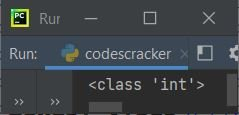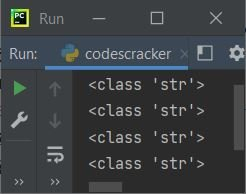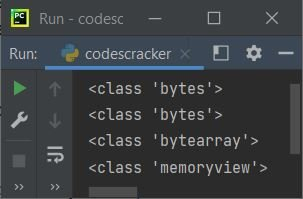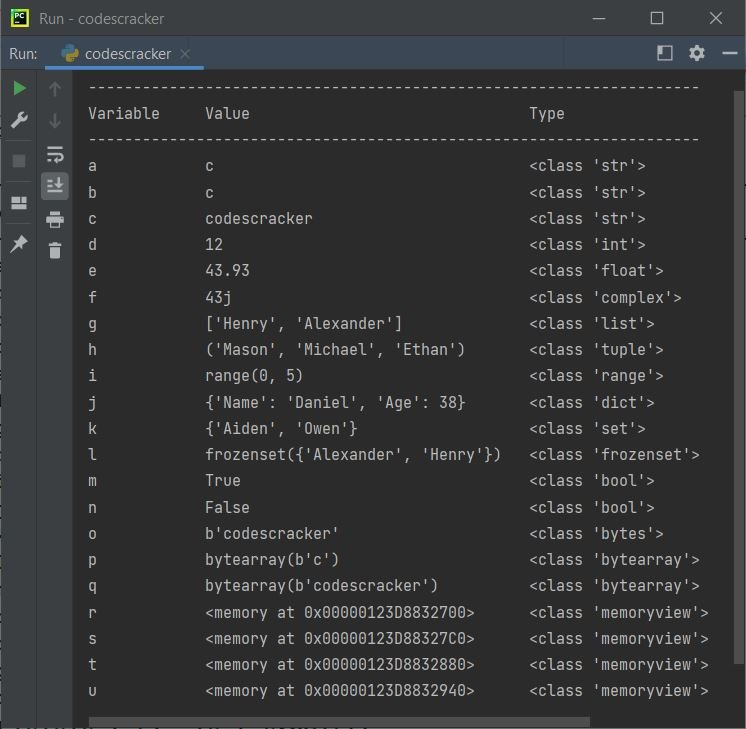# Data Types in Python

Data types in Python are basically attributes of data that tell the compiler or interpreter how to interpret their value. For example, if we have numeric types of data, that means that it can be used to perform arithmetic operations.

## Python 7 Built-in Data Types

The data type represents the type of value and determines how the value can be used in a Python program. Here is the list of seven built-in data types:

1. Text Type
2. Numeric Types
3. Sequence Types
4. Mapping Type
5. Set Types
6. Boolean Type
7. Binary Types

Let's go over each of these seven built-in data types in detail, complete with example programs. But before starting, let's first understand how we can get the type of data that a variable holds.

### How to Determine the Data Type of an Object (Variable)

To find and print the type of data that a variable holds in Python, we need to use the "type()" method. Here is an example program that demonstrates it:

```myvar = 10
print(type(myvar))```

The output produced by the above Python program is shown in the snapshot given below:As you can see from the above output, because the variable "myvar" holds a value of 10, which is an integer value, the output produced says the variable "myvar" is of the class "int."

### Python text data type

The "str" class comes under the category of text data types in Python. This data type holds all the string values. Here is an example:

```myvar = 'c'
print(type(myvar))
myvar = "c"
print(type(myvar))
myvar = "codescracker.com"
print(type(myvar))
myvar = "Hey, What's Up!"
print(type(myvar))```

Here is its sample output:The above program can also be created as:

```a, b, c, d = 'c', "c", "codescracker.com", "Hey, What's Up!"
print(type(a))
print(type(b))
print(type(c))
print(type(d))```

You'll get the same output as the previous program's output.

### Python Numeric Data Types

Under the category of numeric data types, we have:

• int: For example, 123
• float: For example, 3.49
• complex: For example, 95+4j. Here, 95 is a real number, whereas 4 is an imaginary number.

Here is an example program that uses numeric data types. I've used three variables with three different numeric types such as int, float, and complex:

```a, b, c = 123, 3.49, 95.4j
print(type(a))
print(type(b))
print(type(c))```

The output produced by the above program will be:

```<class 'int'>
<class 'float'>
<class 'complex'>```

### Python Sequence Data Types

This is yet another important data type that we must be aware of. This data type deals with values in sequence. A sequence allows us to store multiple values of the same or different types in the same variable. These are the types of sequences listed:

• list: A set of multiple elements (values) separated by commas. All elements must be stored within square brackets, i.e., []. Lists are mutable.
• tuple: Same as list, but all elements must be stored within round brackets, i.e., (). A tuple, on the other hand, cannot be changed, making it immutable.
• range: It generates an immutable sequence. For example, range (5) generates or returns 0, 1, 2, 3, 4.

Note: To learn about these three topics in detail, refer to their separate tutorials.

Let's take an example that uses all these three types of sequence in Python:

```var = [1, "codescracker", True, 23.4, 234]
print(type(var))
var = (1, "codescracker", True, 23.4, 234)
print(type(var))
var = range(10)
print(type(var))```

The output produced by the above program will be:

```<class 'list'>
<class 'tuple'>
<class 'range'>```

### Python Mapping Data Type

The dictionary comes under the category of mapping data types in Python. Each item in the dictionary is a pair of key and value. Since every item (element) is a key-value pair, it falls under the category of mapping data type.

Like lists, the dictionary is also mutable. Here is an example showing the mapping type in Python:

```var = {'day': 'Thursday', 'month': 'September', 'year': '2021'}
print(type(var))
var = {1: 'day', 2: 'month', 3: 'year'}
print(type(var))```

Now the output produced by the above program will be:

```<class 'dict'>
<class 'dict'>```

### Python Set Data Types

Under this data type category, we have basically two types: Here is the list of these two types:

• set: Stores sets of multiple elements separated by commas.All elements must be enclosed by curly braces, i.e. {}. set is mutable.
• frozenset: It also allows for the storage of multiple elements. To create it, first create a list, then use frozenset() to freeze the list. The frozen state is immutable.

Here is an example that uses both types.

```var = {"Liam", "Noah", "Oliver"}
print(type(var))
var = ["Elijah", "William", "Benjamin", "Lucas"]
var = frozenset(var)
print(type(var))```

Now, if you execute this program, then the output produced will exactly look like this:

```<class 'set'>
<class 'frozenset'>```

### Python Boolean Data Type

The boolean data type is used when we need to evaluate one of the two possible values, say, True or False. Here is an example that demonstrates the boolean data type in Python:

```var = True
print(type(var))

var = False
print(type(var))

print(type(True))
print(type(False))```

The output produced by the above Python program demonstrating the boolean data type will be:

```<class 'bool'>
<class 'bool'>
<class 'bool'>
<class 'bool'>```

### Python Binary Data Types

Most of the time, binary data types in Python are used to improve performance. Here is the list of three binary types:

• bytes: Values of the type "bytes" are essentially a sequence of bytes ready to be stored in memory or on disk. Use bytes() to convert anything like strings, numbers, etc. into a sequence of bytes. Or just put strings, numbers, etc. inside b' and '. For example, b'codescracker.com' or bytes("codescracker.com"). Bytes are immutable.
• bytearray: It is an array of bytes. So it is similar to bytes. But unlike bytes, it is mutable.
• memoryview: A memoryview object exposes the C-level buffer interface as a Python object. That is, the memoryview() function, which is used to create a memoryview object, accepts a bytes-type value as an argument.

Here is an example that demonstrates these three binary types.

```var = b"codescracker"
print(type(var))

var = bytes("codescracker", "utf-8")
print(type(var))

var = bytearray(10)
print(type(var))

var = memoryview(bytes(10))
print(type(var))```

The snapshot given below shows the sample output produced by the above Python program:From the above program, consider the following statement:

`var = bytes("codescracker", "utf-8")`

encodes the string "codescracker" using the "utf-8" encoding method.

### Mega Program on Python Data Types

This is the last example program, covering all the data types in Python. First, I initialized all types of values to some variables, then printed the values and types. Let's take a look:

```a = 'c'
b = "c"
c = "codescracker"

d = 12
e = 43.93
f = 43j

g = ["Henry", "Alexander"]
h = ("Mason", "Michael", "Ethan")
i = range(5)

j = {"Name": "Daniel", "Age": 38}

k = {"Owen", "Aiden"}
l = frozenset(g)

m = True
n = False

o = bytes(c, "utf-8")
p = bytearray(b, "utf-8")
q = bytearray(c, "utf-8")
r = memoryview(bytes(d))
s = memoryview(bytes(c, "utf-8"))
t = memoryview(bytearray(d))
u = memoryview(bytearray(c, "utf-8"))

print("--------------------------------------------------------------------")
print("Variable\t Value\t\t\t\t\t\t\t\t Type")
print("--------------------------------------------------------------------")
print("a\t\t\t", a, "\t\t\t\t\t\t\t\t\t", type(a))
print("b\t\t\t", b, "\t\t\t\t\t\t\t\t\t", type(b))
print("c\t\t\t", c, "\t\t\t\t\t\t", type(c))
print("d\t\t\t", d, "\t\t\t\t\t\t\t\t", type(d))
print("e\t\t\t", e, "\t\t\t\t\t\t\t\t", type(e))
print("f\t\t\t", f, "\t\t\t\t\t\t\t\t", type(f))
print("g\t\t\t", g, "\t\t\t", type(g))
print("h\t\t\t", h, "\t\t", type(h))
print("i\t\t\t", i, "\t\t\t\t\t\t", type(i))
print("j\t\t\t", j, "\t\t", type(j))
print("k\t\t\t", k, "\t\t\t\t\t", type(k))
print("l\t\t\t", l, "\t", type(l))
print("m\t\t\t", m, "\t\t\t\t\t\t\t\t", type(m))
print("n\t\t\t", n, "\t\t\t\t\t\t\t\t", type(n))
print("o\t\t\t", o, "\t\t\t\t\t", type(o))
print("p\t\t\t", p, "\t\t\t\t\t", type(p))
print("q\t\t\t", q, "\t\t", type(q))
print("r\t\t\t", r, "\t", type(r))
print("s\t\t\t", s, "\t", type(s))
print("t\t\t\t", t, "\t", type(t))
print("u\t\t\t", u, "\t", type(u))```

There are so many horizontal tabs 😀. The snapshot given below shows the sample output produced by this mega-program on data types in Python:#### More Examples

Python Online Test

« Previous Tutorial Next Tutorial »